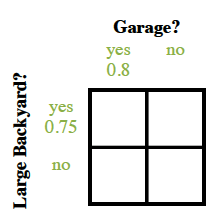### Home > CCA2 > Chapter 11 > Lesson 11.1.1 > Problem11-13

11-13.

$75\%$ of the homes in a small town in England have large backyards, and $80\%$ have garages. Assume that having a garage is independent of whether you have a large backyard.

1. What is the probability a home has neither a garage nor a large backyard? Decide what the two variables in this situation are and make an area model to help answer the question. (See the Math Notes box in this lesson.)Now finish filling out the table. Be sure the review the Math Notes box in this lesson if you need more help.

2. Given that a house has a garage, what is the conditional probability it has a large backyard?

$80\%$ of the homes have garages. Of those, how many have large back yards?

$\frac{?}{0.80}$

$\frac{0.60}{0.80}=?$